# 10:06 MW W 1) The drawing shows three identical springs hanging from the ceiling. Nothing is...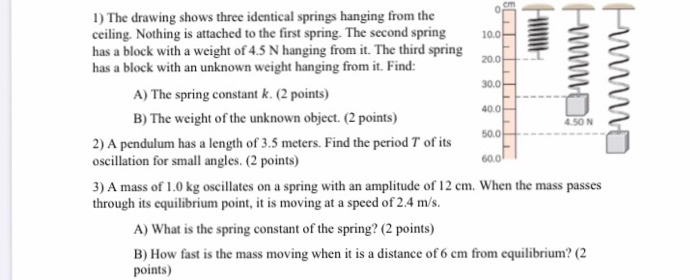10:06 MW W 1) The drawing shows three identical springs hanging from the ceiling. Nothing is attached to the first spring. The second spring has a block with a weight of 4.5 N hanging from it. The third spring has a block with an unknown weight hanging from it. Find: A) The spring constant k. (2 points) 40.04 B) The weight of the unknown object. (2 points) 500 - 2) A pendulum has a length of 3.5 meters. Find the period T of its oscillation for small angles. (2 points) 3) A mass of 1.0 kg oscillates on a spring with an amplitude of 12 cm. When the mass passes through its equilibrium point, it is moving at a speed of 2.4 m/s. A) What is the spring constant of the spring? (2 points) B) How fast is the mass moving when it is a distance of 6 cm from equilibrium? (2 points) 60 01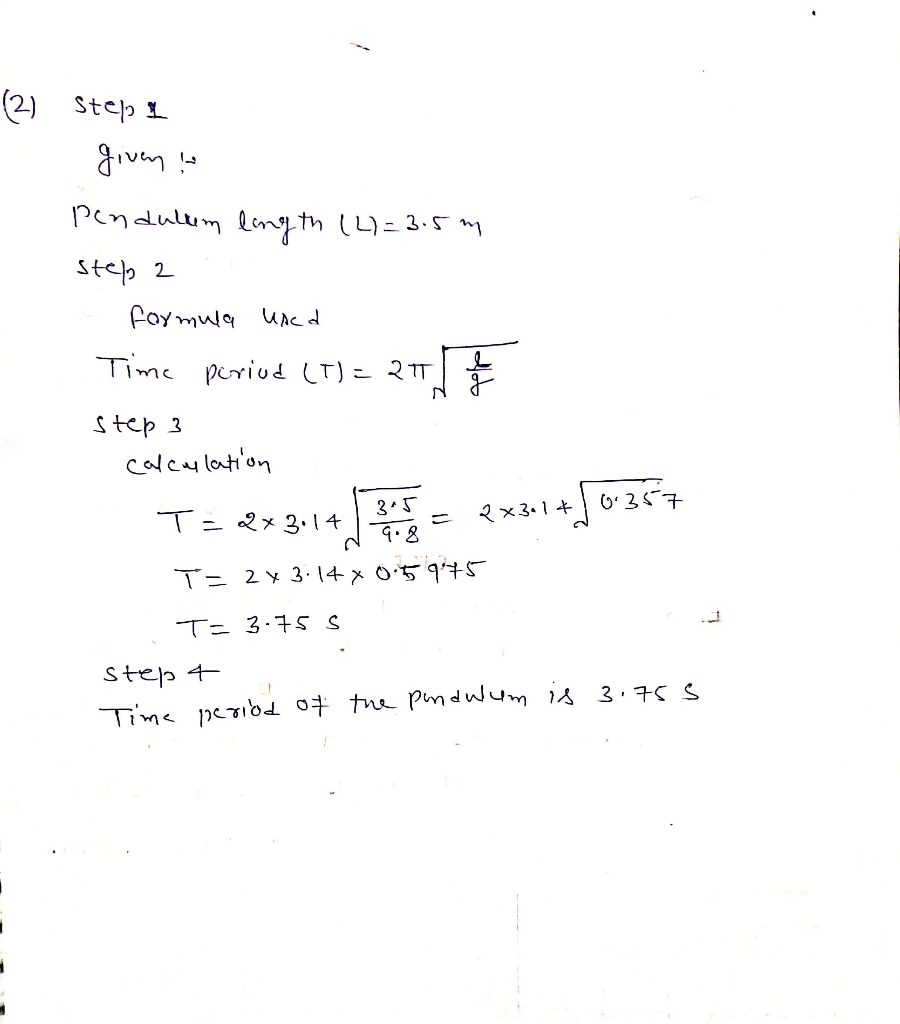#### Earn Coin

Coins can be redeemed for fabulous gifts.

Similar Homework Help Questions
• ### 1) The drawing shows three identical springs hanging from the ceiling. Nothing is attached to the...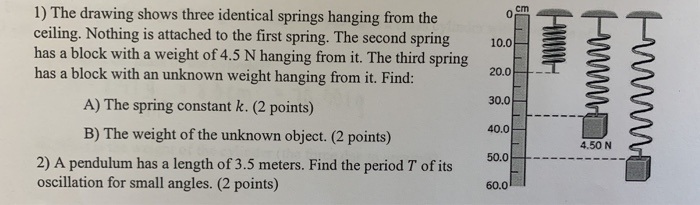1) The drawing shows three identical springs hanging from the ceiling. Nothing is attached to the first spring. The second spring has a block with a weight of 4.5 N hanging from it. The third spring has a block with an unknown weight hanging from it. Find: A) The spring constant k. (2 points) B) The weight of the unknown object. (2 points) 2) A pendulum has a length of 3.5 meters. Find the period T of its oscillation for...

• ### A spring is hanging from a ceiling with 50g mass attached. When the mass is pulled...

A spring is hanging from a ceiling with 50g mass attached. When the mass is pulled down from the equilibrium and released, it oscillates with 0.4s period. The spring constant is equal to

• ### An ideal spring is in equilibrium, hanging from a ceiling with a 1 kg mass at...

An ideal spring is in equilibrium, hanging from a ceiling with a 1 kg mass at the end. At rest, the length of the hanging spring is 10 cm. Then, an additional 5 kg block is added to the spring, causing its length at rest to increase to 13 cm. The 5 kg block is then removed. Starting from rest, when the 5 kg block is removed, the spring begins to oscillate. What will the spring’s velocity be, the third...

• ### A spring is hanging from the ceiling. When a 2 kg mass is hung from the...

A spring is hanging from the ceiling. When a 2 kg mass is hung from the spring, the spring has a total length of 50 cm. When a 5 kg mass is hung from the spring, the spring has a total length of 70 cm. Determine the spring's relaxed length and its spring's spring constant. (You should keep 4 decimals in your values to avoid rounding errors.)

• ### Learning Goal: To use the equations of equilibrium to find unknown forces in two dimensions: understand...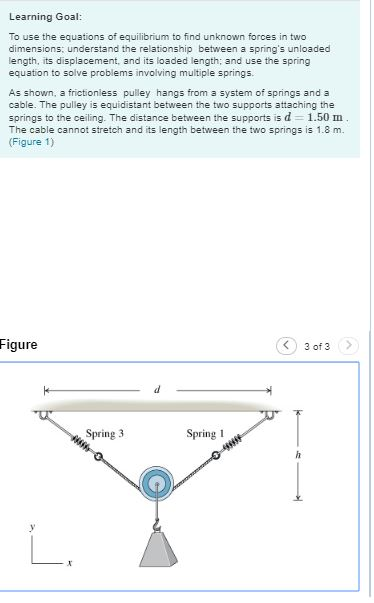Learning Goal: To use the equations of equilibrium to find unknown forces in two dimensions: understand the relationship between a spring's unloaded length, its displacement, and its loaded length and use the spring equation to solve problems involving multiple springs As shown, a frictionless pulley hangs from a system of springs and a cable. The pulley is equidistant between the two supports attaching the springs to the ceiling. The distance between the supports is d = 1.50 m The cable...

• ### 3) A mass of 1.0 kg oscillates on a spring with an amplitude of 12 cm. When the mass passes through its equilibrium...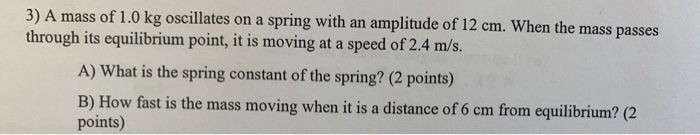3) A mass of 1.0 kg oscillates on a spring with an amplitude of 12 cm. When the mass passes through its equilibrium point, it is moving at a speed of 2.4 m/s. A) What is the spring constant of the spring? (2 points) B) How fast is the mass moving when it is a distance of 6 cm from equilibrium? (2 points)

• ### A gymnast does a one-arm handstand. The humerus, which is the upper arm bone between the...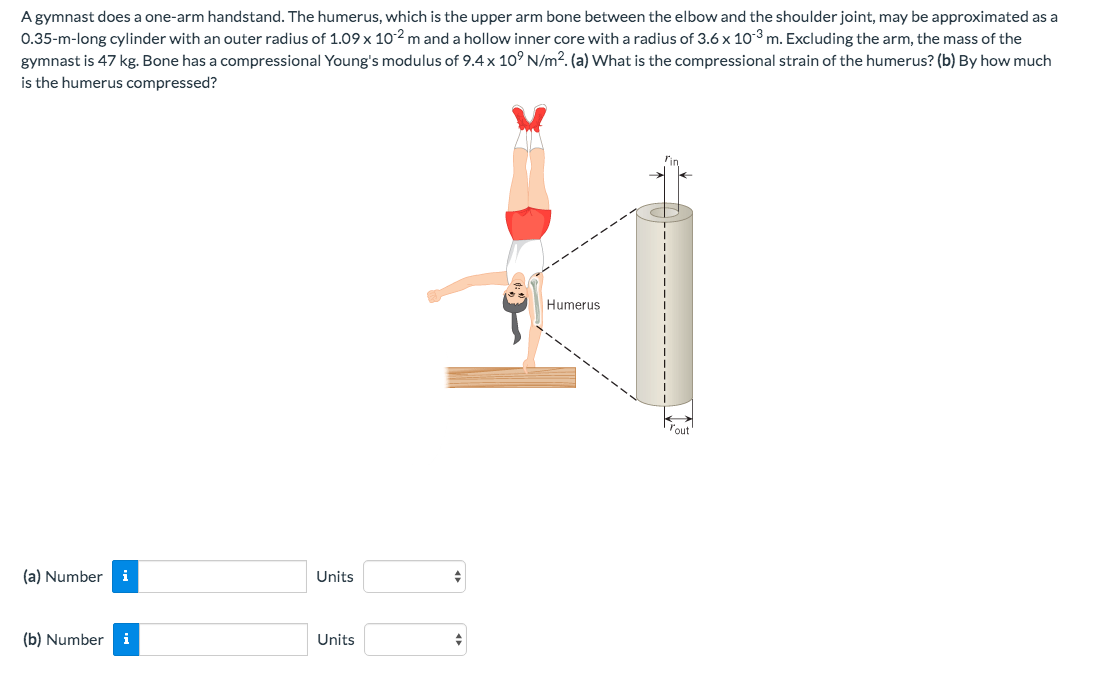A gymnast does a one-arm handstand. The humerus, which is the upper arm bone between the elbow and the shoulder joint, may be approximated as a 0.35-m-long cylinder with an outer radius of 1.09 x 102 m and a hollow inner core with a radius of 3.6 x 10 m. Excluding the arm, the mass of the gymnast is 47 kg. Bone has a compressional Young's modulus of 9.4 x 10' N/m2. (a) What is the compressional strain of the...

• ### Please complete questions #2-3. Show all work. 1. A 250 g mass on a vertical spring...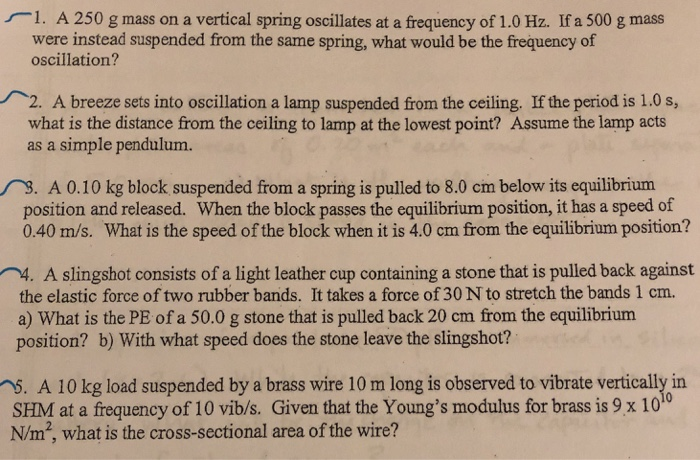Please complete questions #2-3. Show all work. 1. A 250 g mass on a vertical spring oscillates at a frequency of 1.0 Hz. If a 500 g mass were instead suspended from the same spring, what would be the frequency of oscillation? . A breeze sets into oscillation a lamp suspended from the ceiling, If the period is 1.0 s, what is the distance from the ceiling to lamp at the lowest point? Assume the lamp acts as a simple...

• ### Consider a vertical spring with spring constant 23.45 N/m hanging from the ceiling. A small object...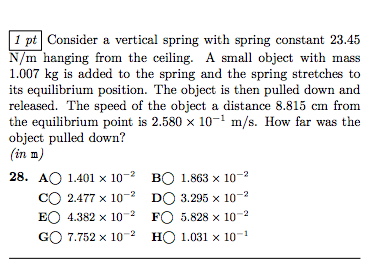Consider a vertical spring with spring constant 23.45 N/m hanging from the ceiling. A small object with mass kg is added to the spring and the spring stretches to its equilibrium position. The object is then pulled down and released. The speed of the object a distance 8.815 cm from the equilibrium point is 2.580 times 10^-1 m/s. How far was the object pulled down? (in m) 1.401 times 10^-2 1.863 times 10^-2 2.477 times 10^-2 3.295 times 10^-2 4.382...

• ### The position of a mass tied to a spring hanging from the ceiling is given by...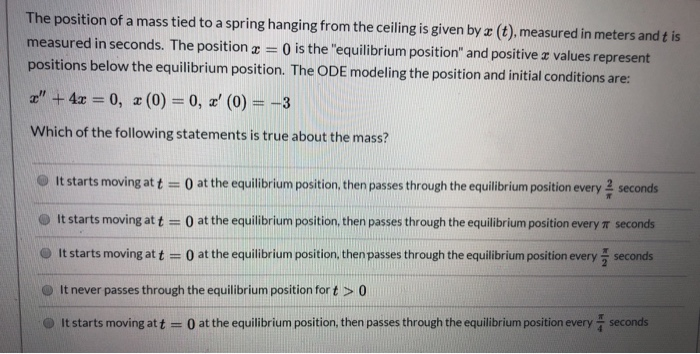The position of a mass tied to a spring hanging from the ceiling is given by x (t), measured in meters and t is measured in seconds. The position I = 0 is the "equilibrium position" and positive e values represent positions below the equilibrium position. The ODE modeling the position and initial conditions are: " + 4x = 0, 2(0) = 0, ' (0) = -3 Which of the following statements is true about the mass? It starts moving...